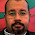## Tuesday, December 7, 2010

### Differential steering challenge problem

I posed a challenge problem in today's guest lecture in the Embedded Systems Programming course.  The challenge is to efficiently control a simple differential steering robot.  The Acumen code below presents both the model of the robot (dsbot), a target object, and a very simple controller.  How well the controller performs is measured in the Main object by the total energy (e) at the end of the simulation.  My score was 161.3.  You are welcome to submit to me the code of an controller that performs better.

The only rule is that you can only send me the code for the controller.  In other words, the code for the robot, target, and the main program has to remain the same.

class dsbot () // Differential steering robot
private x=0; x'=0; y=0; y'=0;
a=0; a'=0 ; v=0
end
x' [=] v * cos(a);
y' [=] v * sin(a);
end

class target () // A simple target moving in a circle
private t=0; t'=0; x=0; y=0 end
t' [=] 1;
x = 2*sin(t/1.5);
y = 2*cos(t/1.5);
end

class controller () // A simple controller
private t =0; t'=0; // local time
ex = 0; ey = 0; // Error in x and y
ia = 0; // Input angle
ov = 0; oap = 0; // Ouput v and a'
end
t' [=] 1;
if t>0.1
t = 0;
// Control code goes here
if (ex*ex + ey*ey) < 1 // Robot is close enough. Slow down
ov = 1
else
ov = 4;
if   (   (ex>0 && cos(ia)<0)
|| (ey>0 && sin(ia)<0))
|| (  (ex<0 && cos(ia)>0)
||(ey<0 && sin(ia)>0))
oap = -10
else
oap = 0
end
end
else
end
end

class Main(simulator)
private r = create dsbot ();
t = create target ();
c = create controller ();
d = 0; e = 0; e'= 0; // Measures of success
end
// Controller see robot
c.ex [=] t.x - r.x;
c.ey [=] t.y - r.y;
c.ia [=] r.a;
// Robot listens to controller
r.a' [=] c.oap;
r.v [=] c.ov;
// Error over time
d [=] sqrt(c.ex*c.ex+c.ey*c.ey);
e' [=] r.v*r.v + 2*d*d
end

1.class controller () // A simple controller
private t =0; t'=0; // local time
ex = 0; ey = 0; // Error in x and y
ia = 0; // Input angle
ov = 0; oap = 0; // Ouput v and a'
end
t' [=] 1;
if t<0.1
t = 0;
// Control code goes here
if (ex*ex + ey*ey) < 2.5 // Robot is close enough. Slow down
ov = 1
else
ov = 4;
if ( (ex>0 && cos(ia)<0)
|| (ey>0 && sin(ia)<0))
|| ( (ex<0 && cos(ia)>0)
||(ey<0 && sin(ia)>0))
oap = -10
else
oap = 0
end
end
else
end
end

1- if we just change the 1 meter distance that we designed to check whether the robot is approaching to target, if we just DECREASE it to 0.25 meter then we get the total energy (e) decreased at the
I discovered that the total energy is oscillating (105 - 185) with the variation of the distance from the target when approaching.
• If we fix this distance to 0.25 meter we get the total energy (e) decreased to 123.
• If we fix this distance to 0.5 meter we get the total energy (e) decreased to 139.
• If we fix this distance to 2.5 meter we get the total energy (e) decreased to 109.
• If we fix this distance to 3.5 meter we get the total energy (e) decreased to 147.
• If we fix this distance to 2 meter we get the total energy (e) decreased to 134.
• Walid Taha’s distance is 1 meter, so we get the total energy (e) of 161.

2- Similarly, if we increase lightly the output velocity V when heading toward target after checking the fixed distance when approaching to target, if we just increase it then we get the total energy (e) decreased. BUT if we increase this velocity to high value, then we get the get the total energy (e) increased.

• If we increase the velocity to 2, then we get the total energy (e) decreased to 129.
• If we increase the velocity to 4, then we get the total energy (e) decreased to 186.
• Walid Taha’s velocity was 1, then we get the total energy (e) of 161.

EIS Master Student
Musab Alhayani

2.Dear Sir
It seems to be perfect,but I was wondering what makes you turn clockwise only,I mean is there any reason that has made you stick to clockwise turn.I need oyur answer to come up with a better code if possible.
BRs.
3.